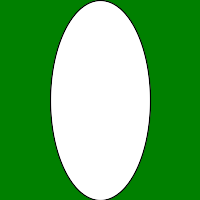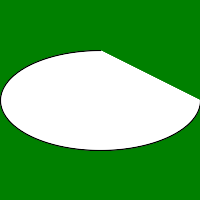# Wand ellipse() function in Python

• Last Updated : 16 Oct, 2021

ellipse() function is used to draw an ellipse on the image. Just similar to drawing circle the ellipse() function requires two pairs of point that is, origin and a pair of (x, y) radius of the ellipse. To draw a partial ellipse, provide a pair of starting & ending degrees as the third parameter.

Syntax :

`wand.drawing.ellipse(origin, radius, rotation)`

Parameters :

Example #1:

## Python3

 `# Import required objects from wand modules``from` `wand.image ``import` `Image``from` `wand.drawing ``import` `Drawing``from` `wand.color ``import` `Color` `# generate object for wand.drawing``with Drawing() as draw:``    ``# set stroke color``    ``draw.stroke_color ``=` `Color(``'black'``)` `    ``# set width for stroke``    ``draw.stroke_width ``=` `1` `    ``# fill white color in arc``    ``draw.fill_color ``=` `Color(``'white'``)``    ``origin ``=` `(``100``, ``100``)``    ``perimeter ``=` `(``50``, ``100``)` `    ``# draw circle using circle() function``    ``draw.ellipse(origin, perimeter)``    ``with Image(width ``=` `200``,``               ``height ``=` `200``,``               ``background ``=` `Color(``'green'``)) as img:``        ``# draw shape on image using draw() function``        ``draw.draw(img)``        ``img.save(filename ``=``'ellipse.png'``)`

Output :Example #2: Drawing partial ellipse using rotation argument

## Python3

 `# Import required objects from wand modules``from` `wand.image ``import` `Image``from` `wand.drawing ``import` `Drawing``from` `wand.color ``import` `Color` `# generate object for wand.drawing``with Drawing() as draw:``    ``# set stroke color``    ``draw.stroke_color ``=` `Color(``'black'``)` `    ``# set width for stroke``    ``draw.stroke_width ``=` `1` `    ``# fill white color in arc``    ``draw.fill_color ``=` `Color(``'white'``)``    ``origin ``=` `(``100``, ``100``)``    ``perimeter ``=` `(``100``, ``50``)``    ``rotation ``=` `(``0``, ``270``)` `    ``# draw circle using circle() function``    ``draw.ellipse(origin, perimeter, rotation)``    ``with Image(width ``=` `200``,``               ``height ``=` `200``,``               ``background ``=` `Color(``'green'``)) as img:``        ``# draw shape on image using draw() function``        ``draw.draw(img)``        ``img.save(filename ``=``'ellipsepartial.png'``)`

Output :My Personal Notes arrow_drop_up